﻿ 风电并网变流器系统中非线性和随机噪声扰动的同步控制研究 Synchronous Control on Wind Power Grid Inverter System with Nonlinear and Stochastic Noise Disturbance

Modeling and Simulation
Vol.05 No.04(2016), Article ID:19101,13 pages
10.12677/MOS.2016.54022

Synchronous Control on Wind Power Grid Inverter System with Nonlinear and Stochastic Noise Disturbance

Jiang Liu1, Long Zhang1, Zhongchuan Jiang2, Yanqing Li1

1Xinjiang University, Urumqi Xinjiang

2Goldwind Science & Technology Co., Ltd., Urumqi Xinjiang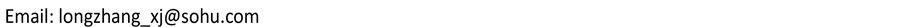Received: Nov. 9th, 2016; accepted: Nov. 26th, 2016; published: Nov. 29th, 2016ABSTRACT

Nonlinear circuits and systems are parts of nonlinear science, which have been central issues in various kinds of research fields. In this paper, we studied the synchronization control of wind power with disturbance of nonlinearity and white noise. By using the Kirchhoff’s law, we obtained the mathematical model of wind power grid side converter system. Meanwhile, an appropriate adaptive synchronization controller was designed. By establishing a suitable Lyapunov function, we validated the effectiveness of synchronous controller. Therefore, we can eliminate the disturbance of nonlinearity and white noise produced by the wind current embedding into the grid. As a result, we can make the current generated by the wind generator synchronize the current in the grid. Finally through Simulink in Matlab simulation, we validated our theoretical results.

Keywords:Nonlinear Perturbation, White Noise, Lyapunov Function, Synchronization, Itô Differential Formula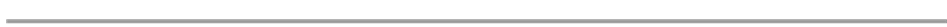1新疆大学，新疆 乌鲁木齐

2金风科技股份有限公司，新疆 乌鲁木齐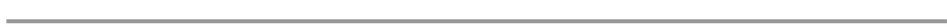1. 引言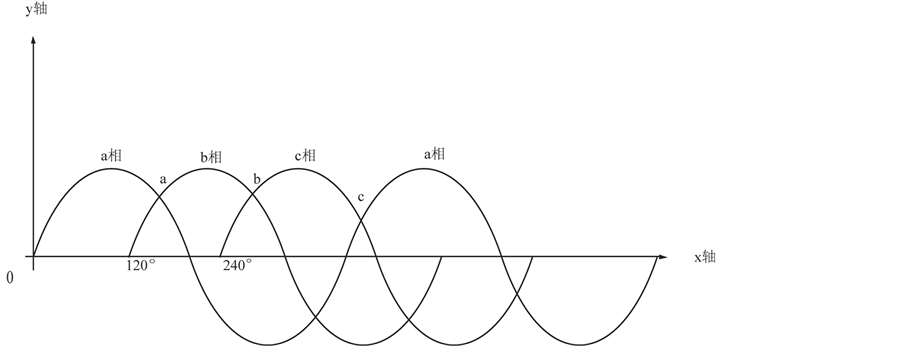Figure 1. Three-phase sinusoidal alternating current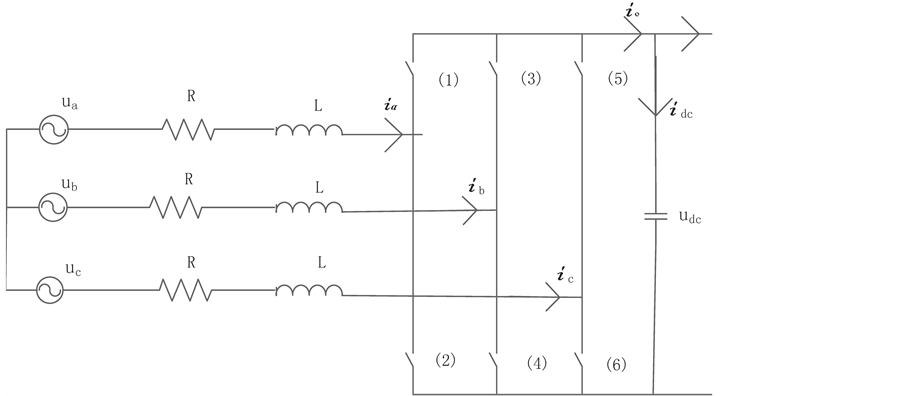Figure 2. The grid side converter main circuit simplified model(1)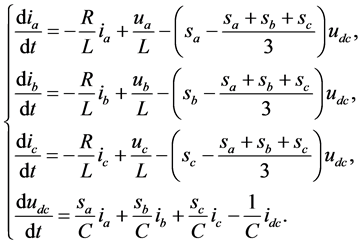(2)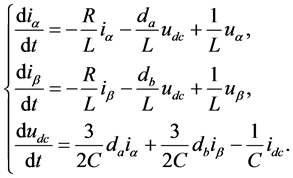(3)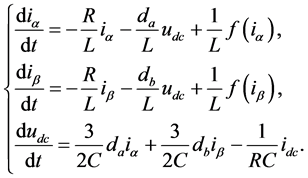(4)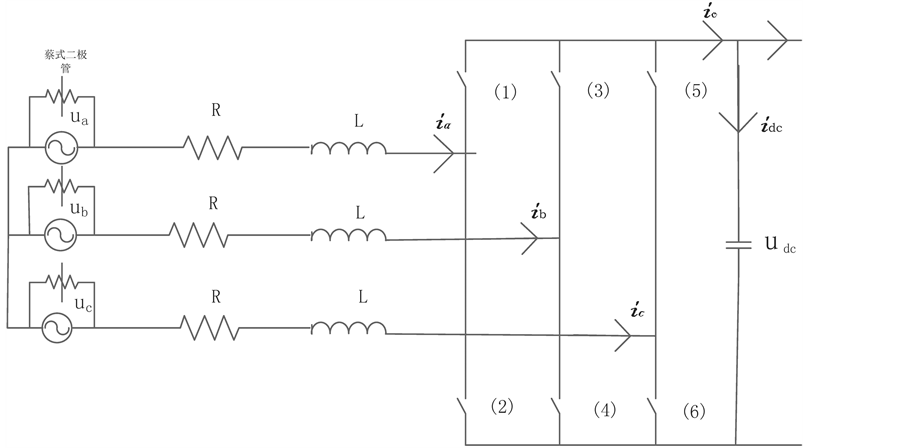Figure 3. Simplified model with nonlinear disturbance in the grid side converter main circuit(6)

2. 预备知识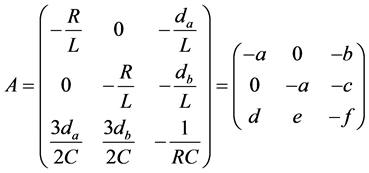(7)(8)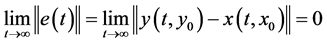(10)(11)是一维布朗运动，且满足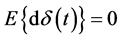，这里是数学期望，并且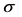是噪声强度。为了方便噪声扰动部分产生误差项，假设响应系统电流强度远大于驱动系统中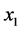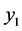电流强度，则响应系统中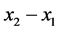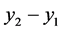可以近似等于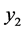。可以用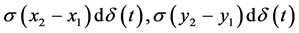近似刻画变流器中开关组不断切换和外部环境等原因对正弦电流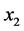造成的噪声扰动。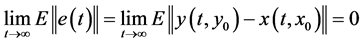3. 主要结果(9)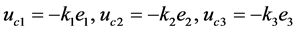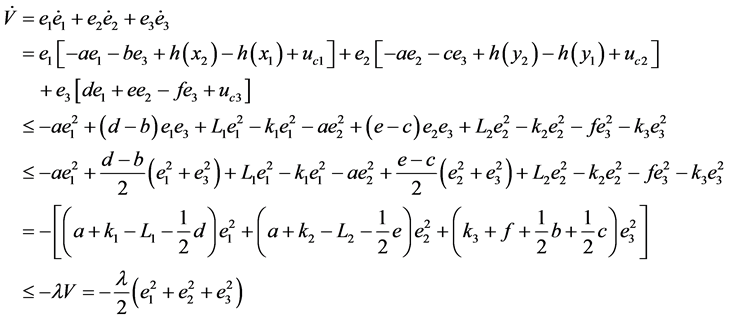(12)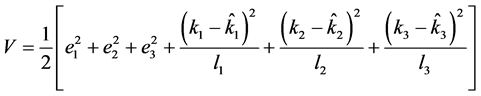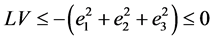4. 仿真及实验验证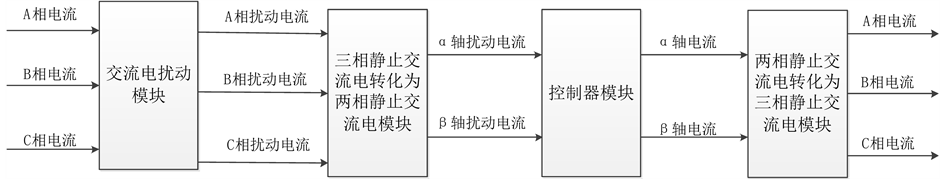Figure 4. Grid side current disturbance control diagram mode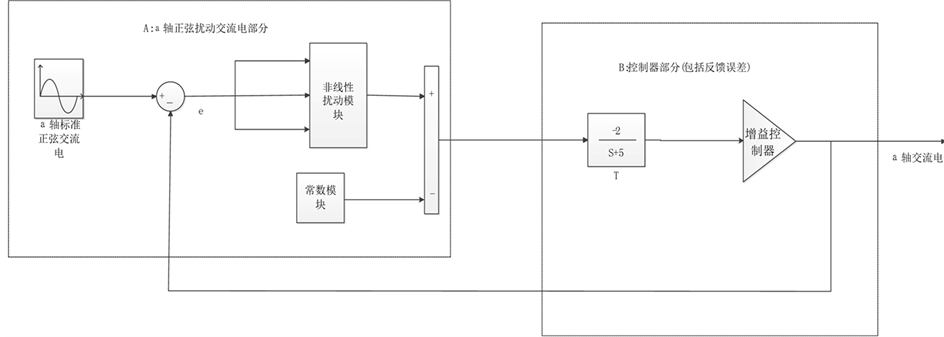Figure 5. Control diagram model of nonlinear disturbanceFigure 6. Nonlinear structural diagram model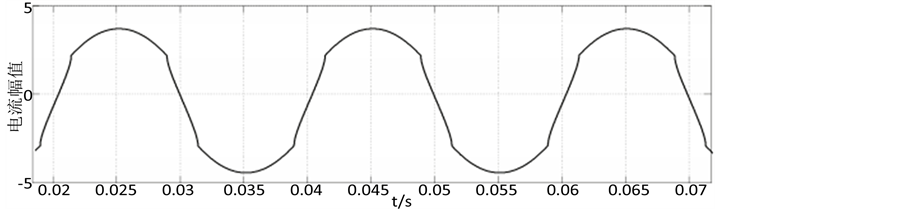Figure 7. Alternating current (ac) of nonlinear disturbance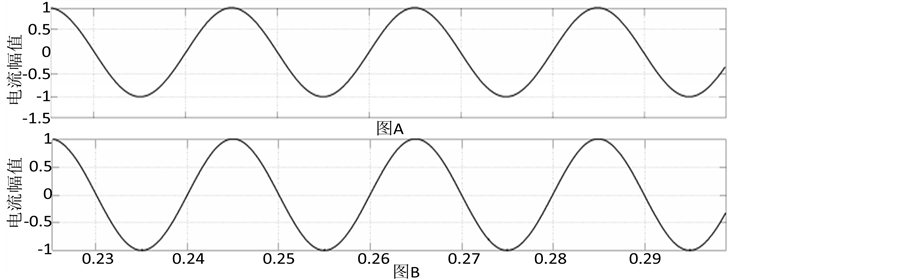Figure 8. Alternating current waveform figureFigure 9. Nonlinear control diagram of the disturbance of noise model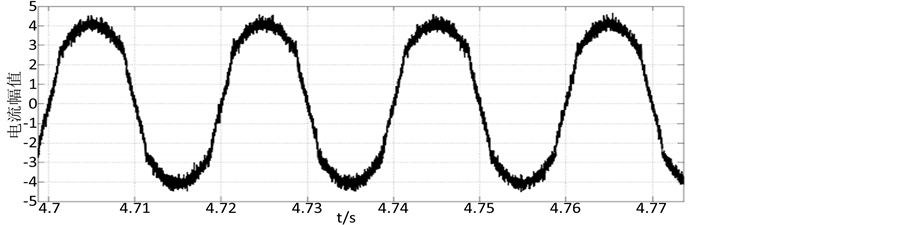Figure 10. The nonlinear noise disturbance of noise intensity σ = 0.05 alternating current waveform figure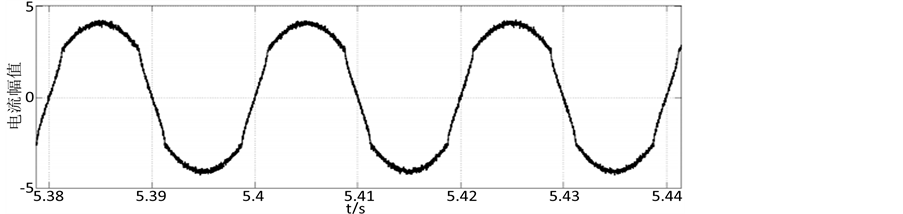Figure 11. The nonlinear noise disturbance of noise intensity σ = 0.012 alternating current waveform figure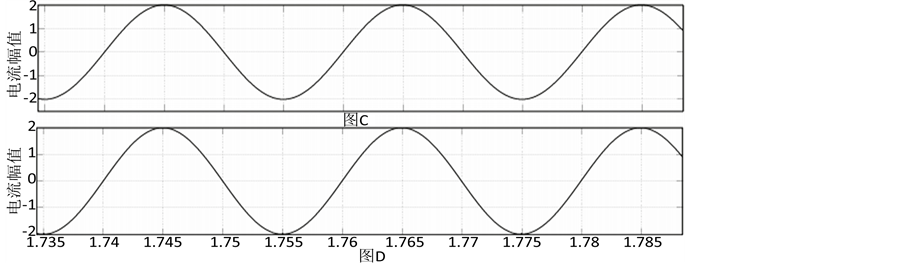Figure 12. Alternating current waveform figure

5. 结论

Synchronous Control on Wind Power Grid Inverter System with Nonlinear and Stochastic Noise Disturbance[J]. 建模与仿真, 2016, 05(04): 170-182. http://dx.doi.org/10.12677/MOS.2016.54022

1. 1. Sainz, L., Mesas, J., Teodorescu, R. and Rodriguez, P. (2010) Deterministic and Stochastic Study of Wind Farm Harmonic Currents. IEEE Transactions on Energy Conversion, 25, 1071-1080. https://doi.org/10.1109/TEC.2010.2045379

2. 2. 张小平, 唐华平, 朱建林, 李白燕. 并网矩阵变换器网侧谐波及稳定性分析[J]. 太阳能学报, 2007, 28(1): 431- 437.

3. 3. 吴捷, 柳明. 非线性控制在电力系统中的应用[J]. 控制理论与应用, 2002, 19(1): 1000-8152.

4. 4. 姚骏, 夏先锋, 陈西寅, 廖勇. 风电并网用全功率变流器谐波电流抑制研究[J]. 中国电机工程学报, 2012, 32(16): 470-476.

5. 5. Sun, Z.Q., Chen, C.Z. and Liang, S.M. (2012) Fault Diagnosis for Large-Scale Wind Turbines. Applied Mechanics and Materials, 210-223, 740-743. https://doi.org/10.4028/www.scientific.net/AMM.220-223.740

6. 6. 庄凯, 廖勇. 直驱式永磁风力发电机组并网变换器关键技术研究[D]: [博士学位论文]. 重庆: 重庆大学, 2012.

7. 7. 张兴, 张崇巍. PWM整流器及其控制策略的研究[D]: [博士学位论文]. 合肥: 合肥工业大学, 2003.

8. 8. 祝贺, 徐建源. 直驱式永磁风力发电机组数学模型及并网运行特性研究[D]: [博士学位论文]. 沈阳: 沈阳工业大学, 2013.

9. 9. 杨志民, 张洁, 马永杰, 摆玉龙, 马胜前. 基于电流传输器的蔡氏混沌电路的设计和实现[J]. 物理学报, 2010, 59(5): 1000-3290.

10. 10. Fang, S.Z., Imkeller, P. and Zhang, T.S. (2007) Global Flows for Stochastic Differential Equations without Global Lipschitz Conditions. The Annals of Probability, 35, 180-205. https://doi.org/10.1214/009117906000000412

11. 11. 牛弘, 张国山. 混沌及超混沌系统的分析、控制、同步与电路实现[D]: [博士学位论文]. 天津: 天津大学, 2014.

12. 12. Zhu, H., Zhou, S.B. and Zhang, J. (2007) Chaos and Synchronization of the Fractional-Order Chua’s System. Chaos, Solitons & Fractals, 21, 120-135.

13. 13. Sun, Y.H. and Cao, J.D. (2007) Adaptive Synchronization between Two Different Noise-Perturbed Chaotic Systems with Fully Unknown Parameters. Physica A: Statistical Mechanics and its Applications, 376, 253-265. https://doi.org/10.1016/j.physa.2006.10.039

14. 14. Friedman (1976) Stochastic Differential Equations and Applications. Academic Press, New York.

15. 15. Mao, X.R. (2002) A Note on the LaSalle-Type Theorems for Stochastic Differential Delay Equations. Journal of Mathematical Analysis and Applications, 268, 125-142. https://doi.org/10.1006/jmaa.2001.7803

16. 16. 胡寿松. 自动控制原理[M]. 第六版. 北京: 科学出版社, 2013: 382-399.